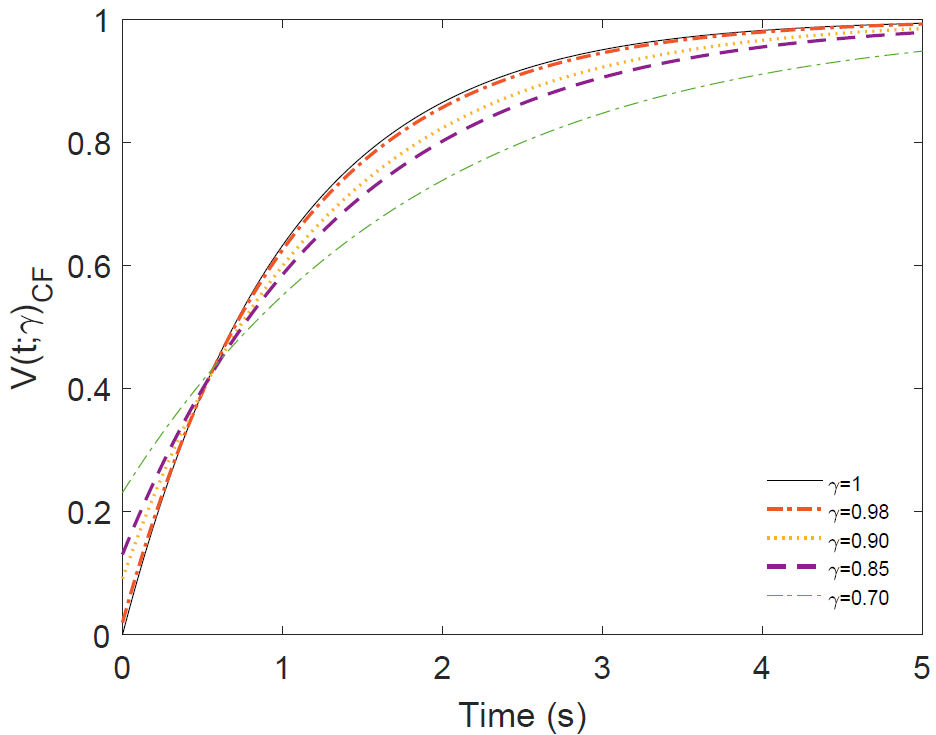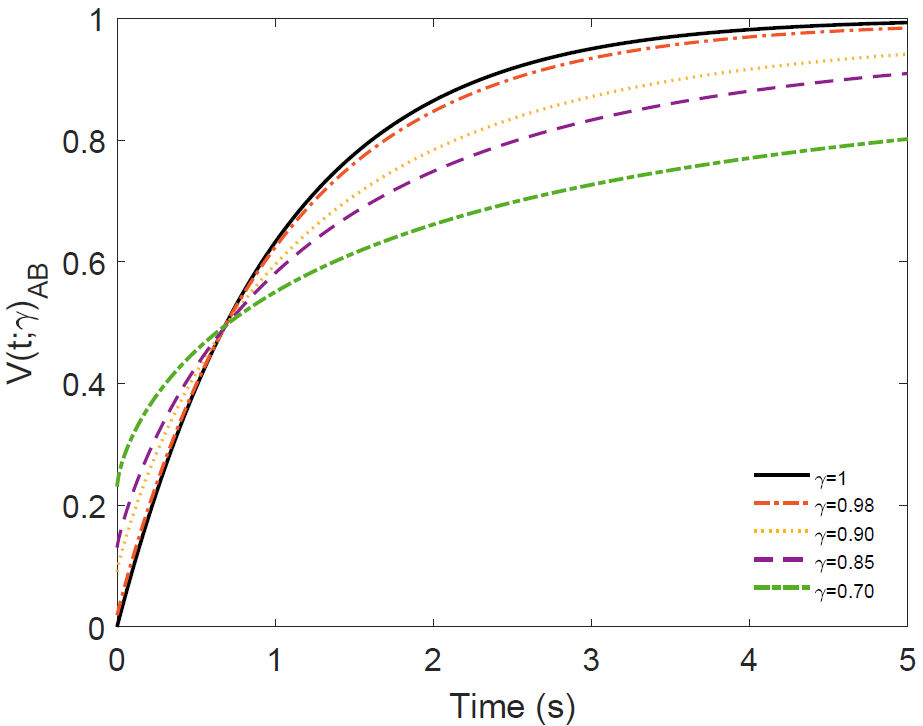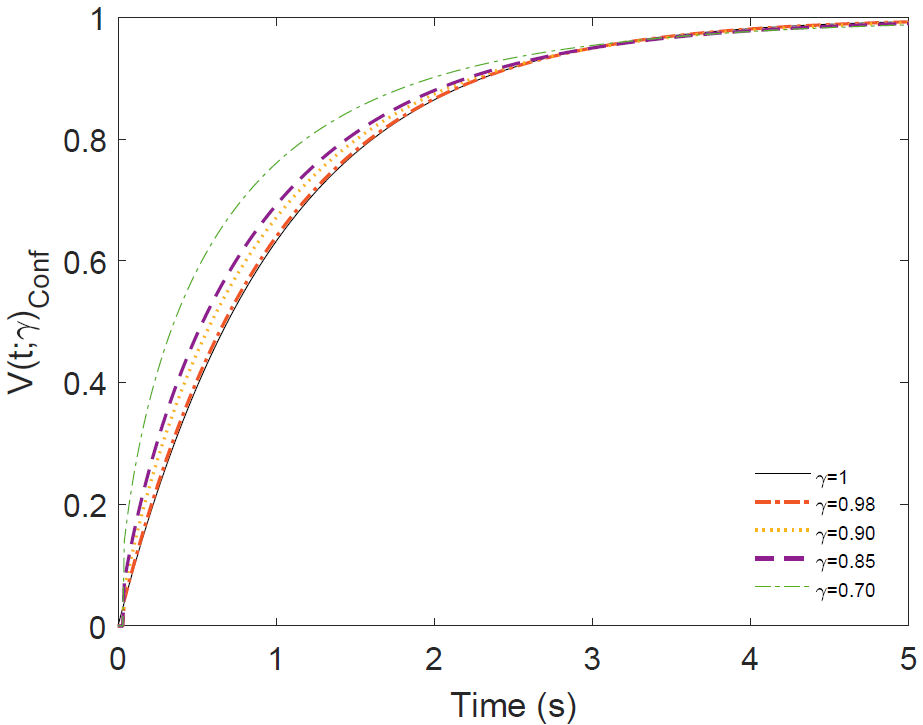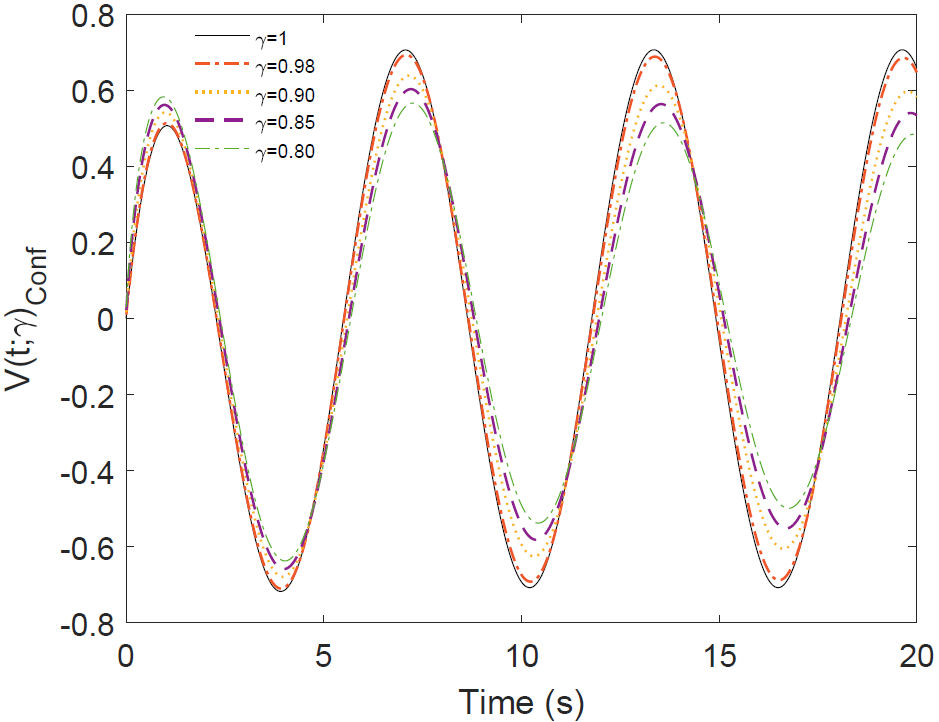## Artigo

•Citado por SciELO
•Acessos

•Similares em SciELO

## versão impressa ISSN 0035-001X

### Rev. mex. fis. vol.64 no.6 México Nov./Dez. 2018  Epub 05-Nov-2019

#### https://doi.org/10.31349/revmexfis.64.647

Research

A comparative analysis of the RC circuit with local and non-local fractional derivatives

a División de Ingenierías Campus Irapuato-Salamanca, Universidad de Guanajuato, Carretera Salamanca-Valle de Santiago, km. 3.5 + 1.8 km. Comunidad de Palo Blanco, Salamanca, Guanajuato, México.

b Escuela de Nivel Medio Superior, Universidad de Guanajuato, Av. Álvaro Obregón, 308, Zona Centro, 37000, León Guanajuato, México.

Abstract

This work is devoted to investigate solutions to RC circuits using four different types of time fractional diferential operators of order 0<𝛾≤1. The fractional derivatives considered are, Caputo, Caputo-Fabrizio, Atangana-Baleanu and the conformable derivative. It is shown that Atangana-Baleanu fractional derivative (non-local), and the conformable (local) derivative could describe a wider class of physical processes then the Caputo and Caputo-Fabrizio. The solutions are exactly equal for all four erivatives only for the case 𝛾=1.

Keywords: Caputo; Caputo-Fabrizio; Atangana-Baleanu; conformable derivative.

PACS: 02.30.Hq; 07.50.Ek

1. Introduction

Fractional calculus (FC), involving derivatives and integrals of non-integer order, is the natural generalization of classical calculus, which during recent decades has become a powerful and widely used method for better modelling and control of processes in many areas of science and engineering [1-5]. The fractional derivative are non-local operators because they are defined using integrals. Therefore, the time fractional derivative contains information about the function at earlier points, thus it possesses a memory effect. Such derivatives consider the history and non-local distributed effects, which are essential for better and more accurate description and understanding of complex and dynamic system behavior [6-18]. Another peculiarity of FC is the inclusion of new degrees of freedom to the system by increasing the information that can be obtained from the nature of the phenomenon in question. Due to the lack of a consistent geometric and physical interpretation of the fractional derivative, several definitions exist  . The most useful definitions are the Riemann-Liouville and Caputo fractional derivatives, however, despite the accurate results obtained with the Riemann-Liouville and Caputo fractional derivatives, they have the disadvantage that their kernel has a singularity at the end point of the interval. To avoid this problem,  proposed the Caputo-Fabrizio derivative (CF). This is a new fractional-order derivative that does not have any singularity. The main advantage of the CF is that the singular power-law kernel is now replaced by a non-singular exponential kernel, which is easier to use in theoretical analysis, numerical calculations and real-world applications. Based on this new derivative, some interesting studies can be found in [21-26]. However, some researchers have concluded that this operator is not a derivative with fractional order, but instead a filter with fractional parameters  . Solutions due to an exponential kernel shows an exponential decay similar to the classical integer order model, therefore, the CF with an exponential kernel has limitations in describing phenomena with non-exponential nature, for example, anomalous relaxation  . To correct this deficiency, two fractional derivatives in the Caputo and Riemann-Liouville sense were defined by Atangana-Baleanu (AB) , based on the generalized stretched Mittag-Leffler function. These new derivatives have been applied to different systems in 30-33.

All definitions of fractional derivatives satisfy the property of linearity, but properties, such as the product rule, quotient rule, chain rule, mean value theorem and composition rule and so on, are lacking in almost all fractional derivatives. These inconsistencies and many more have raised many problems in real applications and have limited the possibilities to explore these fractional calculations. To avoid these difficulties, proposed to extend the ordinary limit definitions of the derivatives of a function called conformable derivative. This conformable derivative has attracted the interest of researchers, as it seems to satisfy all requirements of the standard derivative [35-43].

In this work we try to analyze the difference between the most important definitions of fractional derivatives in a simple system. In particular, we will applied the Caputo (C), Caputo-Fabrizio (CF), Atangana-Baleanu (AB) fractional derivatives and the conformable derivative (conf), in the study of a RC circuit with DC and AC sources.

2. Some definitions of fractional derivatives

The Caputo fractional derivative of order γ is defined by 

aCDtγf(t)=1Γ(1-γ)atf˙(τ)(t-τ)γdτ, (1)

with 0<γ1 and a∈[-∞,t], fH1(a,b), b>a. By changing the kernel (t-τ)-γ with the function e-(γ/1-γ)(t-τ) and 1/(Γ(1-γ)) with (M(γ))/(1-γ), the Caputo-Fabrizio fractional derivative CF  is obtained

where (M(γ)/1-γ) is a normalization function with the property M(0)=M(1)=1. If f(t) is a constant function, then the Caputo (1) and Caputo-Fabrizio derivative (2) are zero. However, in contrast to definition (1), the kernel in (2) does not have singularity at t=τ. This property is of particular interest, because it can describe the full memory effect for a given system. The Laplace transform of the Caputo and Caputo-Fabrizio fractional derivatives 0<γ1 is given by

We have taken the normalization function properties M(0)=M(1)=1 in (4). In , two new fractional derivatives appeared. We will apply one of them, defined as: fH1(a,b), a<b, γ[0,1], then the Atangana-Baleanu fractional derivative in the Caputo sense (AB) is

where Eγ() is the one parameter Mittag-Leffler function defined in

Eγ(z)=n=0znΓ(γn+1). (6)

As can be seen, the Atangana-Baleanu fractional derivative (5) is the natural generalization of the Caputo-Fabrizio derivative (2). The AB derivative has a non-singular and non-local kernel, its Laplace transform is given by ,

where B(γ) has the same properties as in the CF case. Although (1), (2) and (5) are linear operators and possess some fine properties, they do not inherit all the operational behaviours from the typical first derivative, such as product rule, quotient rule, chain rule and semigroup properties. These inconsistencies lead to the development of the local fractional derivative whose most properties coincide with classical integer derivative [34,45-47].

In , the conformable derivative definition is given. It is defined as: Let f:[0,)R being a function. Then, the conformable derivative of the order γ is defined by

Tγf(t)=dγf(t)dtγ=fγ(t)=limϵ0f(t+ϵt1-γ)-f(t)ϵ,0<γ1, (8)

for all t>0. This expression is a possible generalization of the standard definition of derivative. When γ=1 from (8), we obtain

f'=limϵ0f(t+ϵ)-f(t)ϵ.

If f is γ-differentiable in some (0, α), α>0, and limtfγ(t) exist, then we define

fγ(0)=limt0+fγ(t). (9)

The most important properties of this conformable derivative of order γ are given in , we only take the needed expression

Tγf(t)=tn+1-γdn+1dtn+1f(t),γ[n,n+1], (10)

if f(t) is (n+1) differentiable at t>0.

3. The ordinary RC circuit

The equation governing the behavior of the RC circuit is

dV(t)dt+1τV(t)=ϵ(t)τ, (11)

where τ=RC is the time constant of the system measured in seconds, R is the resistance measured in Ohm’s, C is the capacitance measured in Farads, V(t) is the voltage on the capacitor and e(t) is the source. Taking the initial condition as V(0)=0, and assuming a constant source ϵ0, the solution of the equation (11) is

V(t)=ϵ0(1-e-t/τ). (12)

This is the equation that describes the behavior (charging in our case) of a RC circuit with constant source, where its components are ideal. The solution (12) exhibits an exponential decay, when t→∞ the voltage V(t→∞)→ϵ 0, a constant. In the case of an alternating source with angular frequency ω and initial condition V(0)=0, we get

dV(t)dt+1τV(t)=ϵ0τcosωt, (13)

the solution is given by

V(t)=ϵ0ω2τ2+1[cosωt+ωτsinωt-e-tτ]. (14)

4. Fractional RC circuit

Our purpose in this section is to obtain a consisting fractional differential equation corresponding to (11). In  a systematic way to construct fractional differential equations has been proposed. We transform the ordinary derivative in a fractional derivative in the following way

ddt1τ1-γdγdtγ,0<γ1, (15)

where τ=RC is the time constant measured in seconds. The term on the left in (15) has dimensions of s-1, and the one on the right is also. Substituting (15) in (11) we obtain the corresponding fractional differential equation for the RC circuit

dγVdtγ+1τγV(t)=ϵ(t)τγ,0<γ1. (16)

This fractional differential equation has been studied in[49 50]. Our main aim is to analyze this differential equation using different definitions of fractional derivatives including local and non-local, make a comparison of these derivatives and give some conclusions about the way behavior of the solutions.

4.1. RC with Caputo fractional derivative

We consider the fractional differential equation (16) taking into account the Caputo fractional derivative (1), assuming the initial condition be V(0)=0 and ϵ(t)=ϵ0 is a constant. Then, applying the Laplace transform (3), we obtain

sγV(s)+1τγV(s)=ϵ0τγs, (17)

and solving for V(s)=V(s)C, we have

V(s)C=ϵ01τγs(sγ+1τγ). (18)

Using the Laplace inverse transform , we obtain

V(t¯;γ)C=ϵ0[1-Eγ(-t¯γ)],0<γ1, (19)

where t¯=t/τ is a dimensionless parameter. The asymptotic approximations to the Mittag-Leffler function for small t0 and larger t times, in first approximation, are 

Eγ(-tγ)e-tγΓ(1-γ),t0, (20)

Eγ(-tγ)t-γΓ(1-γ),t. (21)

As a consequence, the Mittag-Leffler function interpolates for intermediate time t between the stretched exponential function (20) and the negative power law (21). The stretched exponential function models a very fast decay for small times t, the asymptotic power law is due to very slow decay for large time t, as it can be seen in Fig. (1), showing the behavior of (19), for different values of γ.Figure 1 Plot of the equation (19) for some values of γ corresponding to voltage across the capacitor in the RC circuit of DC.

In Fig. (1), it is observed that in the case of the fractional derivative of Caputo, when the time is between zero and very close to 1 s, the voltage rapidly decays as a stretched exponential depending on the value of γ. After this time, the voltage decays as a negative power depending on the values of γ.

In the case of an oscillatory source, from (16), we have

dγVdtγ+aV=bcosωt, (22)

where a=1/τγ, b=ϵ0/τγ and ω is the frequency of the source. Applying the Laplace transform with V(0)=0 we get V(s)=V(s)C[/p]

V(s)C=bs(s2+ω2)(sγ+a). (23)

We take the highest power of s as a common factor from the denominator, and then we expand the denominator in an alternating geometric series , as a result we obtain

V(s)C=bm,n=0(-1)m+nω2mans(n+1)γ+2m+1. (24)

Then, the inverse Laplace transform gives

V(t;γ)C=ϵ0m,n=0(-1)m+n(ωτ)2mΓ×(tτ)(n+1)γ+2m,0<γ1. (25)Figure 2 Plot of the solution (25) for some values of γ corresponding to voltage across the capacitor in the RC circuit of AC.

4.2. RC with Caputo-Fabrizio fractional derivative

Applying the Caputo-Fabrizio derivative (2) and the Laplace transform (4) to the fractional differential equation (16) with the same conditions V(0)=0,ϵ(t)=ϵ0, we get

V(s)CF=b(γ+As)s(Bs+aγ), (26)

where a=1/τγ, b=ϵ0/τγ, A=1-γ and B=1+a-aγ.

Then, the inverse Laplace transform gives

V(t;γ)CF=ϵ0[1-τγ1-γ+τγe-γ1-γ+τγt],0<γ1. (27)

Then, the CF solution (27) exhibits an exponential decay when t, like as the ordinary integer model. The CF derivative with an exponential kernel (2) has limitations in describing the behaviour of phenomenon with non-exponential nature .

In Fig. (3), similar behavior is shown as in the Caputo case, except that not all the curves start at zero and also change a little after half a second.Figure 3 Plot of the Eq. (27) for some values of γ corresponding to voltage across the capacitor in the RC circuit of DC.

In the case of an oscillating source with angular frequency ω, from (16) and after applying the Laplace transform (4), we have

sV(s)s+γ(1-s)+1τγV(s)=ϵ0sτγ(s2+ω2). (28)

Solving with respect to V(s)=V(s)CF we get

V(s)CF=b(1-γ)s(s+B)(As+aγ)(s2+ω2), (29)

where a=1/τγ, b=ϵ0/τγ, A=1+a(1-γ) and B=(γ/1-γ). As before, taking the highest power of s as as a common factor from the denominator, then expanding the denominator in an alternating geometric series, we obtain

V(s;γ)CF=(1-γ)ϵ0τγ+1-γm,n=0(-1)m+nγmω2nτms2n+m+1+γϵ0τγ+1-γm,n=0(-1)m+nγmω2nτms2n+m+2. (30)

Then, the inverse Laplace transform gives the solution

V(t;γ)CF=(1-γ)ϵ0τγ+1-γm,n=0(-1)m+nγmω2nτmΓt2n+m+γϵ0τγ+1-γm,n=0(-1)m+nγmω2nτmΓt2n+m+1,0<γ1 (31)Figure 4 Numerical simulation of the equation 31) for some values of γ corresponding to voltage across the capacitor in the RC circuit of AC.

5. RC with Atangana-Baleanu fractional derivative

Now, the fractional Eq. (16) is solved using the Atangana-Baleanu fractional derivative (5) and its corresponding Laplace transform (7). For the CC source we have

11-γsγV(s)sγ+γ1-γ+aV(s)=bs, (32)

From here, we get V(s)=V(s)AB,

V(s)AB=Asγs(sγ+C)+AB1s(sγ+C), (33)

where A=(ϵ0(1-γ)/τγ+1-γ), B=(γ/1-γ) and C=(γ/τγ+1-γ). The inverse Laplace transform gives the solution

V(t;γ)AB=ϵ0[1-τγτγ+1-γ×Eγ(-γτγ+1-γtγ)],0<γ1, (34)

where Eγ() is the one-parametric Mittag-Leffler function (6), with the property (20) for t0 and (21) for large time t. In Fig. (5) we have plotted the equation (34) for some values of γ. In the case of Atangana-Baleanu derivative, we observe in Fig. (5) a similar behavior as in the previous cases. However, this behavior is closer to that described by the CF derivative with the only difference that after a certain time the voltage decays much slower than in the two previous cases. This could describe a wider class of physical processes.Figure 5 Numerical simulation of the Eq. (34) for some values of γ corresponding to voltage across the capacitor in the RC circuit of DC.

In the case of an oscillatory source, we have

11-γsγV(s)sγ+γ1-γ+aV(s)=bss2+ω2. (35)Figure 6 Numerical simulation of the Eq. (36) for some values of γ corresponding to voltage across the capacitor in the RC circuit of AC.

Solving for V(s)=V(s)AB and taking the inverse Laplace transform, we get

V(t;γ)AB=ϵ0(1-γ)×m,n=0(-1)m+nω2mγnt2m+nγ+1(1-γ+τγ)n+1Γ[nγ+2(m+1)]+γϵ0m,n=0(-1)m+nω2mγnt2m+(n+1)γ+1(1-γ+τγ)n+1Γ[2m+(n+1)γ+2]. (36)

6. RC with conformable fractional derivative

Finally, replacing the expression (10) in the fractional differential equation (16), for the case 0<γ1,

dγdtγf(t)=t1-γddtf(t), (37)

we have the conformable differential equation for the RC circuit

dVdt+atγ-1V(t)=atγ-1ϵ(t),0<γ1, (38)

where a=(1/τγ). This equation is a linear non-homogeneous ordinary differential equation with variable coefficient of non-integer power 0<γ1, its solution can be found by standard methods. Its solution for the case when the source is a constant ϵ(t)=ϵ0, has the form

V(γ;t)conf=ϵ0[1-exp(-t¯γγ)],0<γ1, (39)

where t¯=(t/τ) is a dimensionless parameter. In this case, we have as solution a stretched exponential function (39). In Fig. (7), we show the plot for different values of γ.Figure 7 This graph shows the equation (39) for some values of γ corresponding to voltage across the capacitor in the RC circuit of DC.

We observe, in Fig. (7), a different behavior as in the three previous cases. The conformable derivative strongly decays the first three seconds, depending on the gamma order, then decays smoothly. Much slower than the three previous cases. Stretched exponential functions are commonly observed in disordered systems [53-59]. The possible origen of the stretched exponential is given in . It is straightforward to note, that in the case γ=1 the stretched exponential function (39) reduces to the ordinary exponential function (12).

In the case of an oscillatory source ϵ(t)=ϵ0cosωt, we have

dVdt+atγ-1V=btγ-1cosωt,0<γ1, (40)

where, a=(1/τγ) and b=(ϵ0/τγ). The numerical solution of equation (40) is given in the Fig. (8).Figure 8 Numerical results of the Eq. (40), corresponding to voltage across the capacitor in the RC circuit of AC.

7. Comparison

In Fig. (9), we have plotted the four solutions given in (19), (27), (34) and (39), for different values of γ. We can observe that the derivatives of (C), (CF) and (AB) have a very similar behavior. They have an almost equal crossing time t1s. Also, it is observed that of these three derivatives, the derivative of Atangana-Baleanu could describe a wider class of physical processes, becuase it is described in terms of Mittag-Leffler function. Finally, the conformable derivative gives as a solution the stretched exponential behavior, the crossing time is tconf3s. This behavior has been observed in a large number of complex physical processes [53-59]. Similar behaviors are observed in the case of alternating current AC, Figs. (2), (4), (6) and (8).Figure 9 Plot of the equations (19), (27), (34) and (39), for different values of γ

8. Conclusion

In this work we have studied the behaviour of the RC electrical circuit for DC and AC sources using four different fractional derivatives. The fractional derivatives were; Caputo (C), Caputo-Fabrizio (CF), Atangana-Baleanu (AB) non-local fractional derivatives and the conformable derivative (conf). The (C) fractional derivative has the disadvantage that their kernel has a singularity at the end point of the interval, then to avoid this problem,  proposed the (CF) derivative. The main advantage of the CF is that the singular power-law kernel is now replaced by a non-singular exponential kernel, which is easier to use in theoretical analysis, numerical calculations and real-world applications. Solutions due to an exponential kernel shows an exponential decay, similar to the classical integer order model, (14) and (27). Therefore, the CF with an exponential kernel has limitations in describing phenomena with non-exponential nature, for example, anomalous relaxation  and stretched exponential relaxation physical processes . To correct this deficiency, two fractional derivatives in the Caputo and Riemann-Liouville sense were defined by Atangana-Baleanu (AB) , based on the Mittag-Leffler function, solutions are of the form (34) with the properties (20) and (21). On the order hand, the conformable derivative was introduced in  . This derivative is a natural extension of the ordinary derivative, as a limit, and has the same properties as the ordinary one. This derivative can describe phenomena, such as relaxation processes in complex systems and so on [53-58]. As far as we know, the conformable derivative had not been applied to the RC circuit.

Theoretically, it was shown that the fractional derivative of Atangana-Baleanu and the conformable derivative could describe a wider class of physical processes then of the Caputo and Caputo-Fabrizio, Fig. 9. The solutions are exactly equal for all four derivatives only for the case γ=1. A next step in the investigation would be to determine, on a practical level, if there are conditions (for DC and AC sources) for which the exposed fractional models represent reality more accurately than the classical models. This may be done in the optimization of the internal parameters of supercapacitors, in process.

Acknowledgments

We would like to thank H.C. Prado Rocha and acknowledge the support provided by DAIP, under the project Optimización de los parámetros internos de un super-capacitor CIIC 135/2018, DICIS-University of Guanajuato. D. Filoteo acknowledges the support provided by CONACyT, under grant 477506.

Referencias

1 C.A. Monje, Y.Q. Chen, B.M Vinagre, D. Xue and V. Feliu, Fractional-Order Systems and Controls, Series: Advances in Industrial control, (Springer 2010). [ Links ]

1 R. Caponetto, G. Dongola, L. Fortuna, I. Petrás, Fractional Order Systems: Modelling and Control Applications, (World Scientific, Singapore, 2010). [ Links ]

2 D. Baleanu, Z.B. Günvenc, J.A. Tenreiro Machado, (Eds) New Trends in Nanotechnology and Fractional Calculus Applications, (Springer 2010). [ Links ]

3 K.B. Oldham, J. Spanier, The fractional calculus, (Academic Press, New York, 1974). [ Links ]

4 I. Podlubny, Fractional differential equations, (Academic Press, New York , 1999). [ Links ]

5 S. Westerlund, Physica Scripta 43 (1991) 174-179. [ Links ]

6 M. Caputo, F. Mainardi, Pure Appl. Geophys. 91 (1971) 134-147. [ Links ]

7 W. Wyss, J. Math. Phys. 27 (1986) 2782-2785. [ Links ]

8 S. Westerlund, IEEE Transactions on Dielectrics and Electrical Insulation (1994) 1 826-839. [ Links ]

9 R. Metzler, J. Klafter, Phys. Reports 339 (2000) 1-77. [ Links ]

10 H. Schiessel, R. Metzler , A. Blumen, F. Nonnenmacher, J. Phys. A: Math. Gen. 28 (1995) 6567-6584. [ Links ]

11 S. Muller, M. Kastner, J. Brummund, V. Ulbricht, Computational Materials Science (2011) 50 2938-2949. [ Links ]

12 A. Giusti, F. Mainardi , Mecanica 51 (2016) 2321-2330. [ Links ]

13 F.C. Meral, T.J. Roytson, R. Magin, Commun. Nonlinear Sci. Numer. Simulat. 15 (2010) 939-945. [ Links ]

14 H. Scher, E.W. Montroll, Phys. Rev. B. 12 (1975) 2455-2477. [ Links ]

15 R. Garrappa, G. Maione, Fractional Prabhakar derivative and applications in anomalous dielectrics: a numerical approach, Theory and Applications of Non-Integer Order System. Ed. Babiar, A., Czornik, A., Klamka, J., Niezabitowski, M., (Springer, pp. 429-439, 2017). [ Links ]

16 R. Garrappa , Commun. Nonlinear Sci. Numer. Simulat 38 (2016) 178-191. [ Links ]

17 F. Mainardi , R. Garrappa , Journal of Computational Physics 293 (2015) 70-80. [ Links ]

18 E.C. de Oliveira, J.A. Tenreiro Machado, Mathematical Problems in Engineering, (2014) Article ID:238459. [ Links ]

19 M. Caputo , M. Fabrizio, Progr. Fract. Differ. Appl. 1 (2015) 73-85. [ Links ]

20 J. Losada, J.J. Nieto, Progr. Fract. Differ. Appl. 1 (2015) 87-92. [ Links ]

22 J.F. Gómez-Aguilar, T. Córdova-Fraga, J.E. Escalante-Martínez, C. Calderón-Ramón, R.F. Escobar-Jiménez, Rev. Mex. Fís. 62 (2016) 144-154. [ Links ]

23 J. Singh, D. Kumar, M. Al Qurashi, D. Baleanu , Open Phys 15 (2017) 35-41. [ Links ]

24 F. Gao, X.J. Yang, Thermal Science 20 (2016) S871-S877. [ Links ]

25 J.M. Cruz-Duarte, J. Rosales-Garcá, C.R. Correa-Cely, A. García-Perez, J.G. Avina-Cervantes, Commun. Nonlinear Sci. Numer. Simulat . 61 (2018) 138-148. [ Links ]

26 M.D. Ortigueira, J.T. Machado, Commun. Nonlinear Sci. Numer. Simulat . 59 (2018) 608-611. [ Links ]

27 S. HonGuan, H. Xiaoxiao, Z. Yong, D. Baleanu , Physica A468 (2017) 590-596. [ Links ]

28 A. Atangana , D. Baleanu , Thermal Science 20 (2016) 763-769. [ Links ]

29 A. Atangana , I. Koca, Chaos Solitons and Fractals 89 (2016) 447-454. [ Links ]

30 J.F. Gómez-Aguilar et al., Entropy 18 (2016) 402. [ Links ]

31 J.F. Gómez-Aguilar , Abdon Atangana, V.F. Morales-Delgado, Int. J. Circ. Theor. Appl. 45 (2017) 1514-1533. [ Links ]

32 B. Saad, T. Alkahtani, Chaos, Solitons and Fractals (2016) 89 547-551. [ Links ]

33 R. Khalil, MAI. Horani, A. Yousef, M.A. Sababheh, J. Comp. Appl. Math. 264 (2014) 65-70. [ Links ]

34 U.N. Katugampola, A new fractional derivative with classical properties, (2014): arXiv: 1410.6535v1. [ Links ]

35 T. Abdeljawad, Journal of Computational Appl. Math 279 (2015) 57-66. [ Links ]

36 Y. Cenesiz, D. Baleanu , A. Kurt, O. Tasbozan, Waves in Random and complex Media 27 (2017) 103-116. [ Links ]

37 D. Zhao, T. Li, J. Math. Computer Sci. 16 (2016) 324-335. [ Links ]

38 M. Al Horani, M. Abu Hammad, R. Khalil , J. Math. Computer Sci. 16 (2016) 147-153. [ Links ]

39 A. Hammad, R. Khalil , Am. J. Comput. and Applied Math 4 (2014) 187-191. [ Links ]

40 A. Atangana , D. Dumitru, A. Alsaedi, Open Math. 13 (2015) 889-898. [ Links ]

41 V.F. Morales-Delgado , J.F. Gómez-Aguilar , M.A. Taneco-Hernández, Int. J. Electron. Commun. 85 (2018) 106-117. [ Links ]

42 A. Oretga, J.J. Rosales, Rev. Mex. Fís. 64 (2018) 172-175. [ Links ]

43 M. Caputo , Geophysical J. International 13 (1967) 529-539. [ Links ]

44 A. Babakhani, V. Daftardar-Gajji, J. Math. Anal. Appl. 270 (2002) 66-79. [ Links ]

45 Y. Chen, Y. Yan, K. Zhang, J. Math. Anal. Appl. 362 (2010) 17-33. [ Links ]

46 A. Atangana , Derivative with a New Parameter: Theory, methods and applications (Elsevier, London 2015). [ Links ]

47 J.J. Rosales , J.F. Gómez, M. Guía, V.I. Tkach, Fractional electromagnetic waves. LFNM, International Conference on Laser and Fiber-Optical Networks Modelling. (2011) 4-8 Sept. Kharkov, Ucraine. [ Links ]

48 F. Gómez, J. Rosales, M. Guía , Centr. Eur. J. Phys. 11 (2013) 1361-1365. [ Links ]

49 H. Ertik, A.E. Calik, H. Sirin, M. Sen, Rev. Mex. Fís. 61 (2015) 58-63. [ Links ]

50 F. Mainardi , Discrete and Continuos Dynamical systems, Series B 19 (2014) 2267-2278. [ Links ]

51 F.Y. Alzoubi, M.K. Alqadi, H.M. Al-Khateeb, S.M. Saadeh, N.Y. Ayoub, Jordan Journal of Physics 5 (2012) 129-134. [ Links ]

52 J.C. Phillips, Rep. Prog. Phys. 59 (1996) 1133-1207. [ Links ]

53 E. Rabani, J.D. Gezelter, B.J. Berne, Phys. Rev. Lett. 82 (1999) 3649-3652. [ Links ]

54 J. Kakalios, R.A. Street, W.B. Jackson, Phys. Rev. Lett. 59 (1987) 1037-1040. [ Links ]

55 R. Chen, Journal of Luminescence 102-103 (2003) 510-518. [ Links ]

56 D. Apitz, J. of Appl. Phys. 97 (2005) 063507. [ Links ]

57 J. Trzmiel, K. Weron, J. Janczura, E. Placzek-Popko, J. Phys: Condens. Matter 21 (2009) 345801. [ Links ]

58 B. Cui, R. Milkus, A. Zaccone, Phys. Letters A. 381 (2017) 416-451. [ Links ]

59 K. Shimakawa, N. Nakagawa, T. Itoh, Appl. Phys. Lett. 95 (2009) 051908. [ Links ]

Received: March 22, 2018; Accepted: May 25, 2018

*e-mail: rosales@ugto.mxThis is an open-access article distributed under the terms of the Creative Commons Attribution License